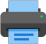Lista de exercícios do ensino médio para impressãoSelect all.
print selected exercises
The length of the arc $\,\stackrel \frown{AB}\,$, as in the picture, is 22 cm and O is the center of the circle. The perimeter of the circle (circumference) is:a)
990 cm
b)
67 cm
c)
176 cm
d)
88 cm
e)

×
What is the radius of the following circle which center is O,
given that:
AB = 3x - 3 and
OA = x + 3.×
Find x in the following cases:
a) s is perpendicular to $\;\overline{AB}\,$b) $\,\overline{PA}\,$ and $\,\overline{PB}\,$ are tangent segments to the circle×
The circles in the picture are externally tangent. The distance between the centers $\,\overline{OC}\,$ is 28 cm and the difference between their radii is 8 cm. Find the length of each radius.answer: 18 cm and 10 cm
×
Knowing that O is the center of the circle, find x in the following cases:
a)b)